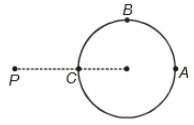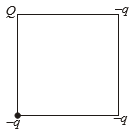A hollow conducting sphere is placed in an electric field produced by a point charge placed at P as shown. Let ${V}_{A},{V}_{B},{V}_{C}$ be the potentials at points A,B and C respectively. Then1. ${V}_{A}<{V}_{B}<{V}_{C}$

2. ${V}_{A}>{V}_{B}>{V}_{C}$

3. ${V}_{C}>{V}_{B}={V}_{A}$

4. ${V}_{A}={V}_{B}={V}_{C}$

Concept Questions :-

Electric potential
High Yielding Test Series + Question Bank - NEET 2020

Difficulty Level:

Four particles each having charge q are placed at the vertices of a square of side a. The value of the electric potential at the midpoint of one of the side will be

1.  0

2.  $\frac{1}{4\pi {ϵ}_{0}}\frac{2q}{a}\left(2+\frac{2}{\sqrt{5}}\right)$

3.  $\frac{1}{4\pi {ϵ}_{0}}\frac{2q}{a}\left(2-\frac{2}{\sqrt{5}}\right)$

4.  $\frac{1}{4\pi {ϵ}_{0}}\frac{2q}{a}\left(1+\frac{1}{\sqrt{5}}\right)$

Concept Questions :-

Electric potential
High Yielding Test Series + Question Bank - NEET 2020

Difficulty Level:

If E be the electric field inside a parallel plate capacitor due to Q and -Q charges on the two plates, then electrostatic force on plate having charge -Q due to the plate having charge +Q will be

(1) -QE

(2) $\frac{-QE}{2}$

(3) QE

(4) $\frac{-QE}{4}$

Concept Questions :-

Capacitance
High Yielding Test Series + Question Bank - NEET 2020

Difficulty Level:

If W be the amount of heat produced in the process of charging an uncharged capacitor then the amount of energy stored in it is

(1) 2W

(2) $\frac{W}{2}$

(3) W

(4) zero

Concept Questions :-

Energy stored in capacitor
High Yielding Test Series + Question Bank - NEET 2020

Difficulty Level:

A metallic sphere of capacitance ${C}_{1}$, charged to electric potential ${V}_{1}$ is connected by a metal wire to another metallic sphere of capacitance ${C}_{2}$ charged to electric potential ${V}_{2}$. The amount of heat produced in the connecting wire during the process is

(1)$\frac{{C}_{1}{C}_{2}}{2\left({C}_{1}+{C}_{2}\right)}{\left({V}_{1}+{V}_{2}\right)}^{2}$

(2) $\frac{{C}_{1}{C}_{2}}{2\left({C}_{1}+{C}_{2}\right)}{\left({V}_{1}-{V}_{2}\right)}^{2}$

(3) $\frac{{C}_{1}{C}_{2}}{{C}_{1}+{C}_{2}}{\left({V}_{1}-{V}_{2}\right)}^{2}$

(4) zero

Concept Questions :-

Energy stored in capacitor
High Yielding Test Series + Question Bank - NEET 2020

Difficulty Level:

The electric potential at the surface of a charged solid sphere of insulator is 20V. The value of electric potential at its centre will be

1.  30V

2.  20V

3.  40V

4.  Zero

Concept Questions :-

Electric potential
High Yielding Test Series + Question Bank - NEET 2020

Difficulty Level:

In the situation shown in the figure, what should be the relation between Q and q so that electric potential at the centre of the square is zero1.  Q = q

2.  Q = 3q

3.  Q = 2q

4.  Q = -3q

Concept Questions :-

Electric potential
High Yielding Test Series + Question Bank - NEET 2020

Difficulty Level:

The capacitance of a parallel plate capacitor is C. If a dielectric slab of thickness equal to one-fourth of the plate separation and dielectric constant K is inserted between the plates, then new capacitance become

1. $\frac{KC}{2\left(K+1\right)}$

2. $\frac{2KC}{K+1}$

3. $\frac{5KC}{4K+1}$

4. $\frac{4KC}{3K+1}$

Concept Questions :-

Dielectrics
High Yielding Test Series + Question Bank - NEET 2020

Difficulty Level:

The electric potential at a point in space due to charge Q is Q V. The value of an electric field at that point will be

1.  $\frac{\mathrm{Q}}{9}×{10}^{12}\frac{\mathrm{N}}{\mathrm{C}}$

2.  $\frac{\mathrm{Q}}{9}×{10}^{15}\frac{\mathrm{N}}{\mathrm{C}}$

3.  $\frac{\mathrm{Q}}{9}×{10}^{9}\frac{\mathrm{N}}{\mathrm{C}}$

4.

Concept Questions :-

Relation between field and potential
High Yielding Test Series + Question Bank - NEET 2020

Difficulty Level:

The electric potential at a point at distance 'r' from a short dipole is proportional to

(1) ${r}^{2}$

(2) ${\mathrm{r}}^{-1}$

(3) ${r}^{-2}$

(4) ${r}^{1}$

Concept Questions :-

Electric potential